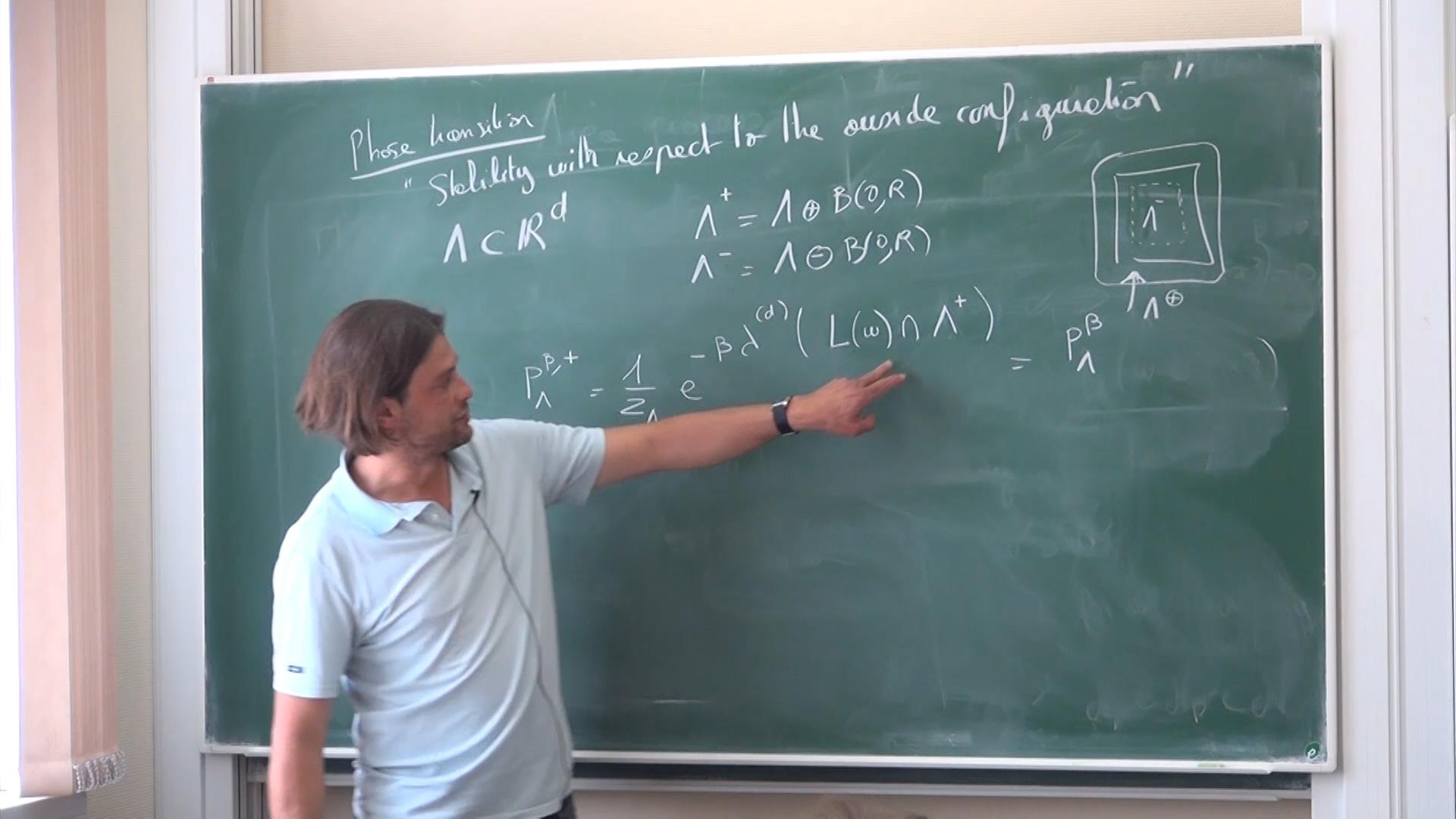# Stochastic Geometry and Statistical Mechanics

Курс
Предмет:
Лектор:Stochastic Geometry and Statistical Mechanics

Prof. David DEREUDRE, University of Lille 1, France.

For many scientists, the modelling and studying of random geometrical structures as tessellations, sets, surfaces or shapes are important challenges. The cellular tissues in Biology, the micro-emulsions in Physics, random simplicial complexes or surfaces in Quantum Gravity, random networks in telecommunication are such important examples. Some models are built by spin systems on lattices (Z^d for example) and are well adapted for a first microscopic description. However some models, at microscopic or macroscopic levels, have to be isotropic and, in a certain sens, more continuous. Spatial continuous models, in general based on PPP or PLP (Poisson Point Process, Poisson Line Process), have been developed to solve these issues. For example, we can mention the Poisson Delauany-Voronoi tessellations, the Boolean models which is just the union of random independent grains centred at the points of a PPP or some modified Poisson line processes (Arak Process, STIT tessellations). All these models are studied in the part of mathematics that we call Stochastic Geometry. Via Gibbs modifcations, interactions based on the morphology of the process are introduced in order to be more relevant for some applications. The models and the tools are now exactly at the interface between Stochastic Geometry and Statistical Mechanics. A lot of rich and interesting problems appear.

The aim of this Minicourse is to present problems between stochastic Geometry and statiscal mechanics.

Bibliography :

1. D. Coupier, D.Dereudre, Continuum percolation for Quermass model, submitted.
2. D.Dereudre, Existence of Quermass processes for non locally stable interaction and non bounded convex grains, Adv. in Appl. Probab. 41 (2009) 664-681. Campbell equilibrium equation and pseudo-likelihood estimation for non-hereditary Gibbs point processes, Bernoulli 15-4, (2009) 1368-1396.
3. D. Dereudre, and H.-O. Georgii, Variational principle of Gibbs measures with Delaunay triangle interaction, Electron. J. prob. 14 (2009) 2438-2462.
4. D. Dereudre, R. Drouilhet and H.-O. Georgii, Existence of Gibbsian point processes with geomety-dependent interactions, Probab. Theory Relat. Fields Vol. 153, No 3-4 (2012) 643-670.
5. Ilya Molchanov, Statistics of the Boolean Model for Practitioners and Mathematicians (1997), Wiley.
6. Jesper Moller, Lectures on Random Voronoi Tessellations (Lecture Notes in Statistics) (1994) Springer-Verlag, New York.
7. D Stoyan, DG Kendall, J Mecke. Stochastic Geometry and its Applications (1995) Wiley.
8. D Ruelle, Statistical Mechanics : Rigorous Results (1969) WA Benjamin.

2